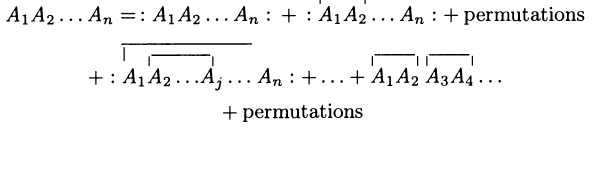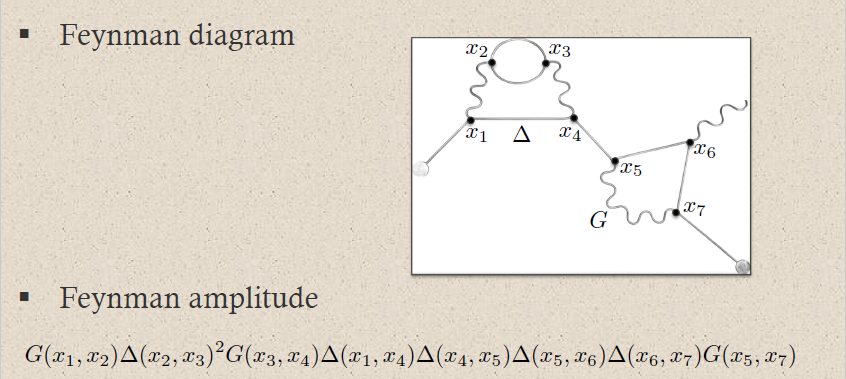# nLab Wick's lemma

Contents

### Context

#### Measure and probability theory

measure theory

probability theory

# Contents

## Idea

Wick’s lemma is a combinatorial formula for the product in associative algebras that appear in the context of free field theory.

From the point of view of path integral quantization, Wick’s lemma is about the moments of Gaussian probability distributions. See at Feynman diagram for more on this.

From the point of view of causal perturbation theory Wick’s lemma expresses the Moyal deformation quantization of a free field theory (Wick algebras) in terms of operator products on.

free field algebra of quantum observablesphysics terminologymaths terminology
1)supercommutative product$\phantom{AA} :A_1 A_2:$
normal ordered product
$\phantom{AA} A_1 \cdot A_2$
pointwise product of functionals
2)non-commutative product
(deformation induced by Poisson bracket)
$\phantom{AA} A_1 A_2$
operator product
$\phantom{AA} A_1 \star_H A_2$
star product for Wightman propagator
3)$\phantom{AA} T(A_1 A_2)$
time-ordered product
$\phantom{AA} A_1 \star_F A_2$
star product for Feynman propagator
perturbative expansion
of 2) via 1)
Wick's lemmaMoyal product for Wightman propagator $\Delta_H$
\begin{aligned} & A_1 \star_H A_2 = \\ & ((-)\cdot (-)) \circ \exp \left( \hbar \int (\Delta_H)^{ab}(x,y) \frac{\delta}{\delta \mathbf{\Phi}^a(x)} \otimes \frac{\delta}{\delta \mathbf{\Phi}^b(y)} \right)(A_1 \otimes A_2) \end{aligned}
perturbative expansion
of 3) via 1)
Feynman diagramsMoyal product for Feynman propagator $\Delta_F$
\begin{aligned} & A_1 \star_F A_2 = \\ & ((-)\cdot (-)) \circ \exp \left( \hbar \int (\Delta_F)^{ab}(x,y) \frac{\delta}{\delta \mathbf{\Phi}^a(x)} \otimes \frac{\delta}{\delta \mathbf{\Phi}^b(y)} \right)(A_1 \otimes A_2) \end{aligned}

From the point of view of BV-quantization Wick’s lemma arises as a consequence of the homological perturbation lemma (Gwilliam, section 2.3).

## Statement

Let $\mathcal{W}$ be the Wick algebra of the free scalar field, hence the space of microcausal observables with product the star product induced by the Wightman propagator:

$\mathcal{W} \;\coloneqq\; \left( PolyObs(E,\mathbf{L})_{mc}, \star_{\Delta_H} \right)$

Then the evident map from $\mathcal{W}$ to linear operators on the Fock space equipped with their operator product $\circ$ is an associative algebra isomorphism onto its image:

$\left( PolyObs(E,\mathbf{L})_{mc}, \star_{\Delta_H} \right) \underoverset{\simeq}{\text{Wick's lemma}}{\hookrightarrow} \left( End(FockSpace), \circ \right)$

Original articles include

The interpretation as an algebra isomorphism to the star product with respect to the Wightman propagator is made explicit in

Textbook accounts include